Electron. J. Differential Equations, Vol. 2019 (2019), No. 115, pp. 1-13.

### Optimal bilinear control for Gross-Pitaevskii equations with singular potentials Kai Wang, Dun Zhao

Abstract:
We study the optimal bilinear control problem of the generalized Gross-Pitaevskii equation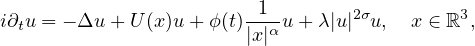where U(x) is the given external potential,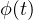is the control function. The existence of an optimal control and the optimality condition are presented for suitable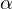and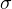. In particular, when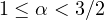, the Frechet-differentiability of the objective functional is proved for two cases: (i)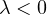,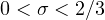; (ii)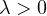,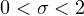. Comparing with the previous studies in , the results fill the gap for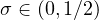.

Submitted February 10, 2019. Published October 13, 2019.
Math Subject Classifications: 35Q55, 49J20.
Key Words: Optimal bilinear control; Gross-Pitaevskii equation; objective functional; Frechet-differentiability; optimal condition.

Show me the PDF file (354 KB), TEX file for this article.Kai Wang School of Mathematics and Statistics Lanzhou University Lanzhou 730000, China email: wkai558@163.com Dun Zhao School of Mathematics and Statistics Lanzhou University Lanzhou 730000, China email: zhaod@lzu.edu.cn